Next: Preserved under action refinement Up: Conclusion Previous: Practical applications

#### Branching bisimulation equivalence has a lower complexity than any other abstract semantic equivalence used in concurrency theory.

No abstract semantic equivalence used in concurrency theory is as easy to decide as branching bisimulation congruence. For context-free process without silent actions bisimulation equivalence is the only equivalence in the linear time - branching time spectrum surveyed in VAN GLABBEEK (1990b) that is decidable at all (cf. GROOTE & HÜTTEL (1994) and CHRISTENSEN, HÜTTEL & STIRLING (1995)). For finite-state processes, (strong) bisimulation equivalence can be decided in polynomial time, whereas most other equivalences in the linear time - branching time spectrum are PSPACE-complete (cf. KANELLAKIS & SMOLKA (1990)).

In GROOTE & VAANDRAGER (1990) an algorithm is presented for deciding branching bisimulation equivalence between finite-state processes, with (time) complexity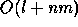. Here l is the size of Act, n is the number of nodes in the investigated process graphs and m the number of edges. The fastest algorithm for weak bisimulation equivalence up till then had time complexity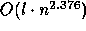. In general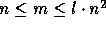, so it depends on the density of edges in a graph which algorithm is faster. In a trial implementation of the scheduler of MILNER (1980), reported in GROOTE & VAANDRAGER (1990), branching bisimulation turned out to be much faster. Furthermore, it turned out that in such automatic verifications the space complexity was a much more serious handicap then the time complexity (the weak bisimulation tools suffered from lack of memory already when applied to processes with 15.000 states). The space complexity of the algorithm of GROOTE & VAANDRAGER (1990) is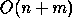, which is less than the space complexity of the weak bisimulation algorithm. Recently, BOUALI (1992) proposed another algorithm for weak bisimulation that has the same time complexity as the branching bisimulation algorithm. An trial with Milner's scheduler shows that it is somewhat slower, but with a constant factor only. The space complexity of BOUALI's algorithm is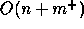, where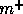is the number of edges after taking the t-transitive closure, obtained by adding an edge p --t-> q whenever there is a nonempty path p ===> q. This improves the space complexity of the old algorithm for weak bisimulation, but is not quite as good as the branching bisimulation algorithm.Next: Preserved under action refinement Up: Conclusion Previous: Practical applications

Rob van Glabbeek